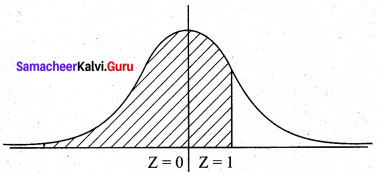Students can download 12th Business Maths Chapter 7 Probability Distributions Ex 7.3 Questions and Answers, Samacheer Kalvi 12th Business Maths Book Solutions Guide Pdf helps you to revise the complete Tamilnadu State Board New Syllabus and score more marks in your examinations.

Tamilnadu Samacheer Kalvi 12th Business Maths Solutions Chapter 7 Probability Distributions Ex 7.3

Question 1.
Define Normal distribution.
Solution:
A random variable X is said to follow a normal distribution with parameters µ (mean) and σ2 (variance) if its probability density function is given by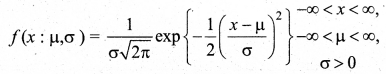Question 2.
Define Standard normal variate.
Solution:
A random variable Z = $$\frac{X-\mu}{\sigma}$$ is called a standard normal variate with mean 0 and standard deviation 1 (i.e.) Z ~ N (0, 1). Its probability density function is given by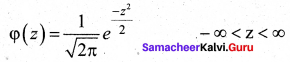Question 3.
Write down the conditions in which the Normal distribution is a limiting case of the binomial distribution.
Solution:
The Normal distribution is a limiting case of Binomial distribution under the following conditions:

• n, the number of trials is infinitely large, i.e. n → ∞
• neither p (or q ) is very small.

Question 4.
Write down any five chief characteristics of Normal probability curve.
Solution:
Chief Characteristics of the Normal Probability Curve are as follows:

• The curve is bell-shaped and symmetrical about the line x = µ.
• Mean, median and mode of the distribution coincide.
• The total area under the normal curve is equal to unity.
• For a given µ and σ, there is only one normal distribution.
• The Points of inflexion are given by x = µ ± σQuestion 5.
In a test on 2,000 electric bulbs, it was found that bulbs of a particular make, was normally distributed with an average life of 2,040 hours and a standard deviation of 60 hours. Estimate the number of bulbs likely to burn for
(i) more than 2,150 hours
(ii) less than 1,950 hours
(iii) more 1,920 hours but less than 2,100 hours.
Solution:
Let X be the numbers of hours for which the bulbs are in use. It is given that X is normally distributed with mean 2040 hours and a standard deviation of 60 hours, (i.e) X ~ N (2040, 602)
(i) P (X > 2150)
We change to the standard normal variate.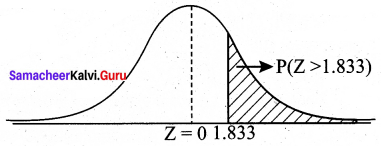The total area to the right of Z = 0 is 0.5.
The area between Z = 0 and 1.833 is 0.4664 (from tables)
So P (Z > 1.833) = 0.5 – 0.4664 = 0.0336
The number of bulbs likely to bum for more than 2150 hours is 2000 × 0.0336 = 67.2 ~ 67

(ii) We want P (X < 1950)
= P($$\frac{\mathrm{X}-\mu}{\sigma}<\frac{1950-2040}{60}$$)
= P(Z < -1.5)
The area between Z = -1.5 and Z = 0 is same as area between Z = 0 and Z = 1.5.
From the tables, area between Z = 0 and Z = 1.5 is 0.4332
P(Z < -1.5) = 0.5 – 0.4332 = 0.0668
Hence the number of bulbs likely to bum for less than 1950 hours is 2000 × 0.0668 = 133.6 ~ 134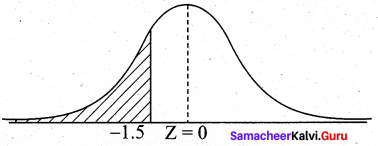(iii) We want P(1920 < X < 2100)
When X = 1920,
$$Z=\frac{X-\mu}{\sigma}=\frac{1920-2040}{60}=-2$$
When X = 2100,
$$\mathrm{Z}=\frac{2100-2040}{60}=\frac{60}{60}=1$$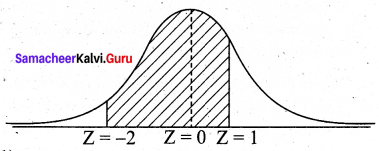So P(1920 < X < 2100) = P(-2 < Z < 1)
= P(-2 < Z < 0) + P(0 < Z < 1)
= P (0 < Z < 2) + P (0 < Z < 1)
= 0.4772 + 0.3413
= 0.8185
Hence the number of bulbs likely to bum for more than 1920 hours but less than 2100 hours is 2000 × 0.8185 = 1637.Question 6.
In a distribution, 30% of the items are under 50 and 10% are over 86. Find the mean and standard deviation of the distribution.
Solution:
Let X be the normal random variable denoting the number of items in the distribution.
It is given that 30% of items are under 50
⇒ P (X < 50) = 30% = 0.3.
Also, 10% are over 86
⇒ P (X > 86) = 10% = 0.1.
We have to find µ and σ.
Representing the given data diagrammatically,
Where Z1 = $$\frac{50-\mu}{\sigma}$$ and Z2 = $$\frac{86-\mu}{\sigma}$$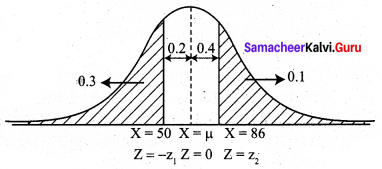From the diagram,
P (-Z1 < Z < 0) = 0.2
By symmetry P (0 < Z < Z1) = 0.2
Z1 = 0.525 (from the normal table)
Hence -0.525 = $$\frac{50-\mu}{\sigma}$$
(i.e.) 50 – µ = -0.525σ …….. (1)
Again P(0 < Z < Z2) = 0.4
Z2 = 1.28
Hence $$\frac{86-\mu}{\sigma}$$ = 1.28
(or) 86 – µ = 1.28σ ……. (2)
Solving (1) and (2)
50 – µ = -0.525σ
86 – µ = 1.28σ
Subtracting,
36 = 1.28σ + 0.525σ
36 = 1.805σ
σ = 19.94
Using this in (2),
86 – µ = 1.28 (19.94)
86 – µ = 25.52
µ = 60.48
Hence the mean of the distribution is 60.48 and standard deviation is 19.94.

Question 7.
X is normally distributed with mean 12 and SD 4. Find P(X ≤ 20) and P(0 ≤ X ≤ 12).
Solution:
Given X ~ N (12, 42), (i.e) mean (µ) = 12 and s.d (σ) = 4.
P(X ≤ 20)
= P($$\frac{X-\mu}{\sigma} \leq \frac{20-12}{4}$$)
= P(X ≤ 2)
Now P(Z ≤ 2) = P(Z ≤ 0) + P(0 ≤ Z ≤ 2)
= 0.5 + 0.4772
= 0.9772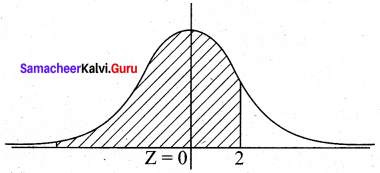P(0 ≤ X ≤ 12)
= P($$\frac{0-12}{4} \leq \frac{X-\mu}{\sigma} \leq \frac{12-12}{4}$$)
= P (-3 ≤ Z ≤ 0)
= P(0 ≤ Z ≤ 3)
P(0 ≤ Z ≤ 3) = 0.49865 (from normal tables)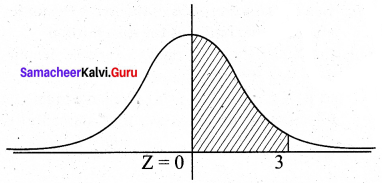Question 8.
If the heights of 500 students are normally distributed with mean 68.0 inches and standard deviation of 3.0 inches, how many students have height
(a) greater than 72 inches
(b) less than or equal to 64 inches
(c) between 65 and 71 inches.
Solution:
Given X is the normal random variables denoting the height of the students with mean µ = 68 and s.d (σ) = 3.
(a) To find P(X > 72)
= P(Z > $$\frac{72-68}{3}$$)
= P(Z > $$\frac{4}{3}$$)
= P(Z > 1.33)
Now P(Z > 1.33) = 0.5 – P(0 < Z < 1.33)
= 0.5 – 0.4082
= 0.0918
Hence number of students whose height is greater than 72 inches is 500 × 0.0918 = 45.9 ~ 46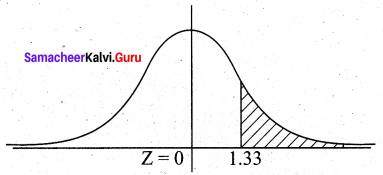(b) To find P(X ≤ 64)
= P(Z ≤ $$\frac{64-68}{3}$$)
= P(Z ≤ -1.33)
By Symmetry,
P(Z ≤ -1.33) = P(Z ≥ 1.33) = 0.0918 (see before section)
Hence number of students whose heights are less than or equal to 64 inches is 0.0918 × 500 = 46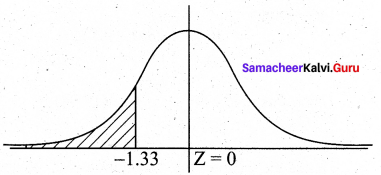(c) To find P (65 < X < 71)
= P($$\frac{65-68}{3}$$ < Z < $$\frac{71-68}{3}$$)
= P(-1 < Z < 1)
= P(-1 < Z < 0) + P(0 < Z < 1)
= 2P(0 < Z < 1) {By symmetry}
= 2 (0.3413)
= 0.6826
Hence number of students with height between 65 and 71 inches is (500) (0.6826) = 341.3 ~ 342.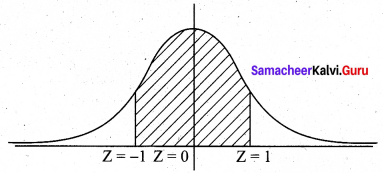Question 9.
In a photographic process, the developing time of prints may be looked upon as a random variable having the normal distribution with a mean of 16.28 seconds and a standard deviation of 0.12 second. Find the probability that it will take less than 16.35 seconds to develop prints.
Solution:
Let X be the normal random variable denoting the developing time of the prints.
Given that mean µ = 16.28 and s.d σ = 0.12
To find P(X < 16.35)
When X = 16.35, $$\mathrm{Z}=\frac{\mathrm{X}-\mu}{\sigma}=\frac{16.35-16.28}{0.12}$$
Z = 0.58
So P(X < 16.35) = P(Z < 0.58)
Now P(Z < 0.58) = 0.5 + P(0 < Z < 0.58)
= 0.5 + 0.2190
= 0.7190
Thus the probability that it will take less than 16.35 seconds to develop prints is 0.719.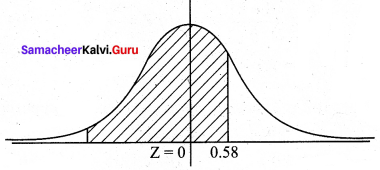Question 10.
Time taken by a construction company to construct a flyover is a normal variate with mean 400 labour days and a standard deviation of 100 labour days. If the company promises to construct the flyover in 450 days or less and agree to pay a penalty of ₹ 10,000 for each labour day spent in excess of 450. What is the probability that
(i) the company pays a penalty of at least ₹ 2,00,000?
(ii) the company takes at most 500 days to complete the flyover?
Solution:
Let X be the normal variate denoting the number of labour days.
Given mean µ = 400 and s.d σ = 100
(i) The company pays penalty of ₹ 2,00,000 at the rate of ₹ 10,000 per each extra labour day.
No. of extra days = $$\frac{2,00,000}{10,000}$$ = 20
So the probability that company pays a penalty of atleast ₹ 2,00,000 is probability that labour days should be atleast 450 + 20 = 470 days, (i.e.) P (X ≥ 470)
Now P(X ≥ 470) = P(Z ≥ $$\frac{470-400}{100}$$) = P (Z ≥ 0.7)
P(Z ≥ 0.7) = 0.5 – P(0 ≤ Z ≤ 0.7) = 0.5 – 0.258 = 0.242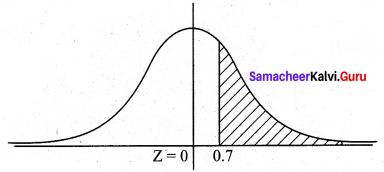(ii) P (X ≤ 500) = P(Z ≤ $$\frac{500-40}{100}$$) = P (Z ≤ 1)
P(Z ≤ 1) = 0.5 + P (0 ≤ Z ≤ 1) = 0.5 + 0.3413 = 0.8413
Thus the probability that the company takes at most 500 days to complete the flyover is 0.8413.